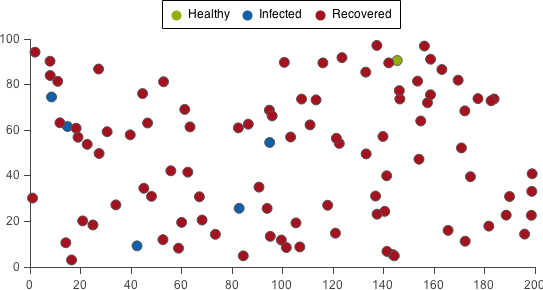# Disease Dynamics (ABM)

This tutorial describes how to construct a model of an infectious disease using Insight Maker. Agent-Based Modeling techniques are used in the construction of this model. Before starting the tutorial, make sure you have familiarized yourself with how to create primitives and run models.
1. Create a new State named Healthy.
2. Create a new State named Infected.
3. Create a new State named Recovered.
4. The model diagram should now look something like this: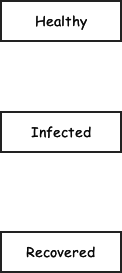States represent the condition someone is in. So in our model a person can either be healthy, infected, or recovered from the infection. Now, lets add transitions that move a person from state to state.
5. Create a new Transition going from the primitive Healthy to the primitive Infected. Name that transition Infection.
6. Create a new Transition going from the primitive Infected to the primitive Recovered. Name that transition Recovery.
7. The model diagram should now look something like this: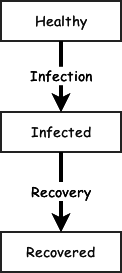Please note that in this model someone who is recovered cannot become sick again. They have gained immunity to the disease.

Now that the model structure has been designed, let's add equations and configure the primitives.
8. Change the Initial Active property of the primitive Healthy to true.
When a state is active, it means a person is in that state. By setting Healthy to start true, we have the person start in the healthy state.
9. Change the Trigger Type property of the primitive Infection to Probability.
10. Change the Value/Equation property of the primitive Infection to 0.3.
11. Change the Trigger Type property of the primitive Recovery to Probability.
12. Change the Value/Equation property of the primitive Recovery to 0.2.
Using the Probability type for the transition trigger means that the person has a fixed probability of transitioning from one state to the next each year.
13. Run the model. Here are sample results: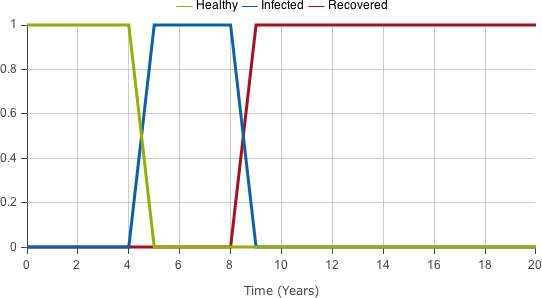Don't worry if your results do not look exactly like this. This is a stochastic model so each time we run it, we will get different results. Let's give it a try.
14. Run the model. Here are sample results: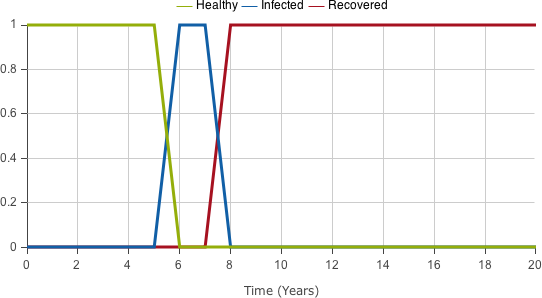15. Run the model. Here are sample results: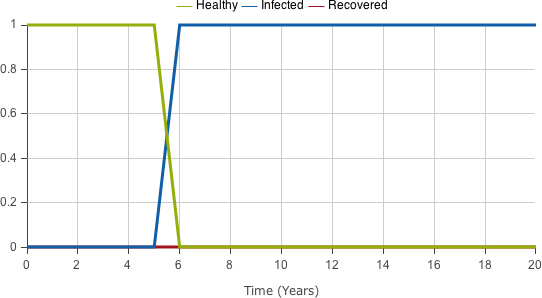When a state takes on the value 1 it means the person is in the state. We can see how the person moves from healthy, to infected, to recovered in these three different simulation runs. Right now we are only simulating a single person. Let's extend our model to simulate a population of people.
16. Create a new Folder named Person. The folder should surround the primitives Healthy, Infected, Recovered, Infection and Recovery.
17. The model diagram should now look something like this: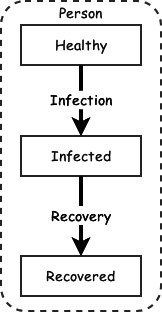18. Change the Behavior property of the primitive Person to Agent.
19. Create a new Agent Population named Population.
20. Change the Agent Base property of the primitive Population to Person.
21. The model diagram should now look something like this: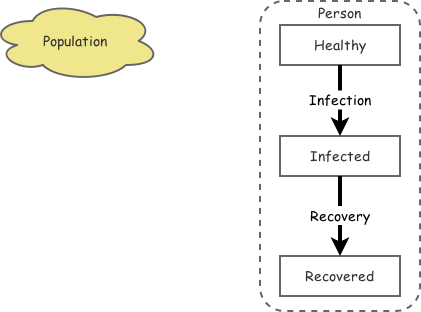22. Run the model. Here are sample results: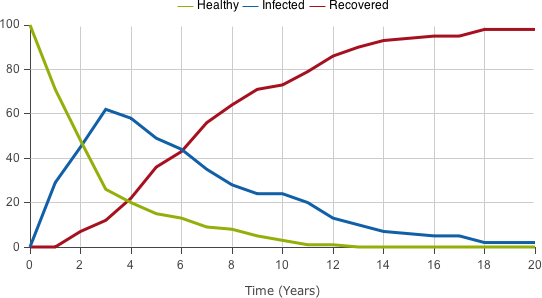That looks pretty good! We can see the disease gradually spread through our population of 100 people.

However, right now the probability of a person getting sick is independent of the other people in the model. Let's change the model so the more sick people there are, the higher the likelihood that a healthy person will become sick.
23. Create a new Variable named Percent Infected.
24. Create a new Link going from the primitive Population to the primitive Percent Infected.
25. Create a new Link going from the primitive Percent Infected to the primitive Infection.
26. The model diagram should now look something like this: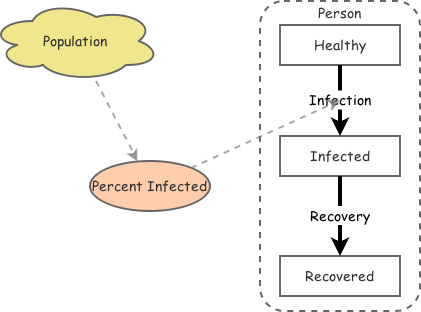Now let's configure the value of Percent Infected and change the Infection transition to use it.
27. Change the Equation property of the primitive Percent Infected to Count(FindState([Population], [Infected]))/PopulationSize([Population]).
This equation uses the FindState function to select all the people in the Population primitive who are in the Infected state. It then divides the count of those people by the total size of the population.
28. Change the Value/Equation property of the primitive Infection to [Percent Infected], and the Recalculate property of the primitive Infection to Yes.
The Recalculate property causes the infection rate to be recalculated and updated at every time step of the simulation.
29. Run the model. Here are sample results: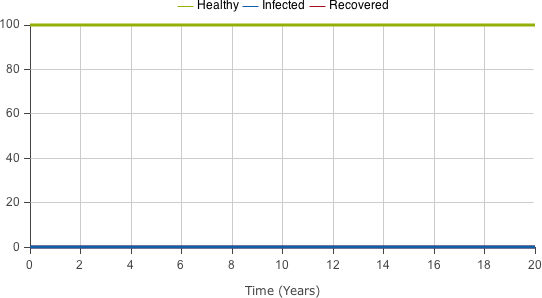Nothing happened because we don't have anyone to start the infection. Let's seed the model with a single initial infected person.
30. Change the Initial Active property of the primitive Infected to Index(Self) == 1.
31. Change the Initial Active property of the primitive Healthy to Index(Self) <> 1.
Each of the people in the population is given an unique Index. The first agent will have an index of 1, the second an index of 2, and so on. Self always refers to the current agent. These equations will set the first agent created to start in the Infected state while all the other agents will start in the Healthy state.
32. Run the model. Here are sample results: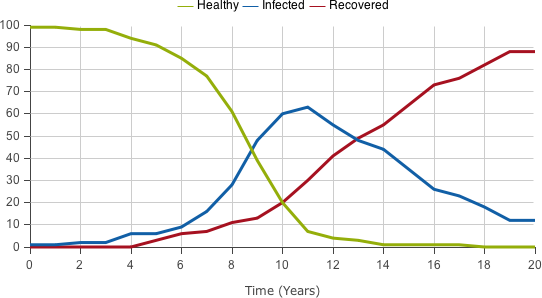Since this is a stochastic model, each time you run it, you will get slightly different results. Let's give it a try.
33. Run the model. Here are sample results: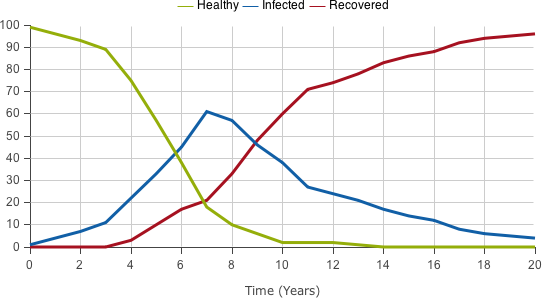34. Run the model. Here are sample results: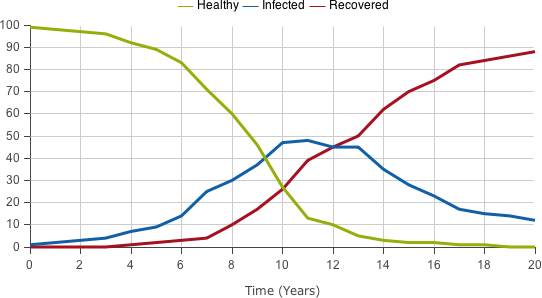That works great! We now have a full Agent-Based Model of disease.

We can also configure the display type to be a map of the people in the model in a two-dimensional geography. Right now, people are placed randomly, but we could configure their locations or make them move around in response to different factors. We could also change the infection rate so the infection spread geographically.
35. Run the model. Here are sample results: